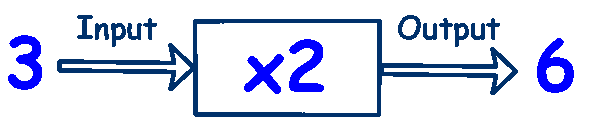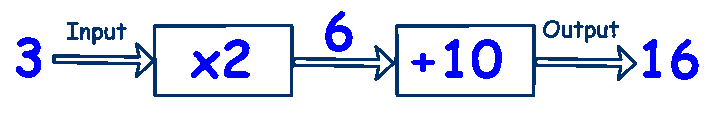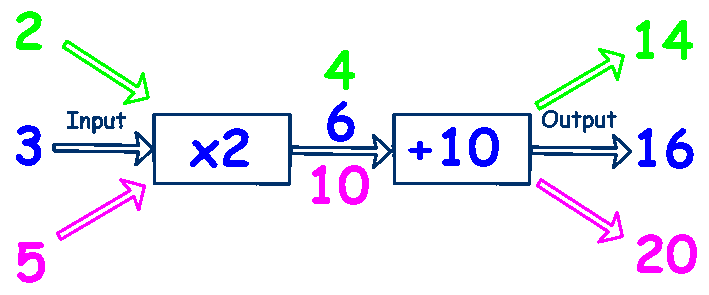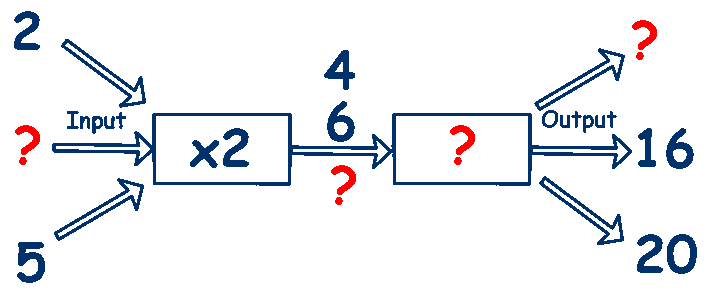# Function Machines

A Function Machine is a diagram that represents a machine that takes an input, applies a rule such as a set of operations and delivers the answer as an output.

Your task is either to determine the input, the output or whatever operations take place inside the machine. Let's look at an example.There is an input, 3, a set of operations within the machine, x 2, and an output, 6

A typical question could be:The number 2 enters the machine, is multiplied by 4, giving a result 2 x 4 = 8

You could be asked to find the input, the output or the operation.

There may be more than one operation in the machine,In the 11 Plus, you can see function machines with three inputs and outputs,And you may see a question like this,You just need to work through it logically, an input from the top left is the output from the top right. An input from the bottom left is the output from the bottom right.

Function machines can be drawn in many different way, but the idea is the same.

Try these worksheets from Mathsblog

or you could practice with these online Function Machine